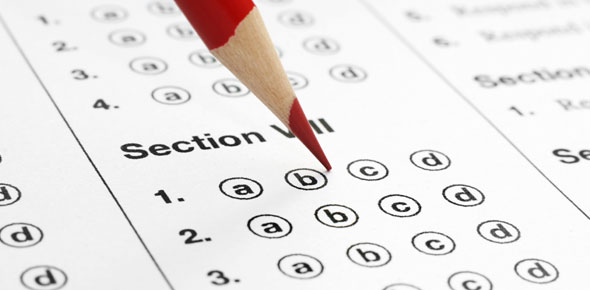# Achr131 Module Final Review

50 Questions | Total Attempts: 773SettingsPossible Multiple Choice Questions

• 1.
A split-phase has a _____ winding and a _____winding.
• A.

Start, run

• B.

Start, common

• C.

Common, run

• D.

Run, common

• 2.
A split-phase motor can use a _____to energize and de-energize the start winding.
• A.

Potential relay

• B.

Current relay

• C.

Centrifugal switch

• D.

All of the above

• 3.
A current magnetic relay opens and closes its contacts based on the _____.
• A.

Current in the start winding

• B.

Voltage across the start winding

• C.

Voltage across the run winding

• D.

Current in the run winding

• 4.
A permanent split capacitor (PSC) motor that is running and up to speed will have the start winding _____ .
• A.

Energized

• B.

De-energized

• C.

In series with the run winding

• D.

Removed from the circuit

• 5.
A split-phase motor that has a current relay and a start capacitor is called a _____motor.
• A.

Capacitor Start, Capacitor Run (CSCR)

• B.

Capacitor Start, Induction Run (CSIR)

• C.

Induction Start, Induction Run (ISIR)

• D.

Permanent Split Capacitor (PSC)

• 6.
When a Capacitor Start, Capacitor Run (CSCR) motor initially starts, what is the total capacitance acting on the motor?  (Assume the run capacitor is 5 microfarads and the start capacitor is 20 microfarads.)
• A.

• B.

• C.

• D.

• 7.
When a Capacitor Start Induction Run (CSIR) motor is running and up to speed, what is the total capacitance acting on the motor?  (Assume the run capacitor is 5 microfarads and the start capacitor is 20 microfarads.)
• A.

• B.

• C.

• D.

• 8.
The starting device usually used on single phase, fractional horsepower compressor motors is the _____.
• A.

Potential relay

• B.

Current relay

• C.

Centrifugal switch

• D.

None of the above

• 9.
A motor's speed is determined by _____.
• A.

Size

• B.

Voltage

• C.

Amperage

• D.

Number of motor poles

• 10.
Start windings are placed _____the run windings.
• A.

Outside

• B.

Inside

• C.

Between

• D.

In series with

• 11.
The start windings have _____.
• A.

Fewer turns than the run windings

• B.

More turns than the run windings

• C.

The same number of turns as the run windings

• D.

Larger diameter wire than the run windings

• 12.
When a general duty split-phase motor reaches approximately 75% of its operating speed, the start winding is de-energized by a _____.
• A.

Circuit board

• B.

Capacitor

• C.

Centrifugal switch

• D.

Dual voltage switch

• 13.
Capacitors wired in series with the starting winding provide _____.
• A.

Longer starting time

• B.

Greater starting torque

• C.

An aid in starting three phase motors

• D.

More efficient operation at full load speed

• 14.
• A.

Has little starting torque

• B.

Has excessive starting torque

• C.

Has greater efficiency than most split-phase motors

• D.

Will start against a heavy starting load

• 15.
Three phase motors have _____.
• A.

Three start and three run windings for each phase

• B.

One start and one run winding for each phase

• C.

Run windings only

• D.

A start switch in each phase circuit

• 16.
The positive-temperature-coefficient start device is a type of _____.
• A.

Capacitor

• B.

Thermistor

• C.

Switching device

• D.

Diode

• 17.
A permanent split-capacitor motor has a _____.
• A.

Start and run capacitor

• B.

Start capacitor only

• C.

Run capacitor only

• D.

Split phase capacitor

• 18.
When referring to motors, to what does the "insulation class" relate?
• A.

The noise level of the motor

• B.

The type of base on which the motor is mounted

• C.

The normal operating temperature of the motor

• D.

Both a and b

• 19.
Why are explosion-proof motors not normally used on standard equipment?
• A.

They are too large.

• B.

They are too noisy.

• C.

They are too expensive.

• D.

They cannot be replaced.

• 20.
The motor that should be used in a potentially wet area is a(n) _____motor.
• A.

Enclosed

• B.

Drip proof

• C.

Explosion proof

• D.

Open

• 21.
Which of the following voltages are not safe for a 208-V motor to operate?
• A.

170 volts

• B.

220 volts

• C.

238 volts

• D.

Both a and c

• 22.
A sleeve bearing would typically be used in a motor that operates with a _____.
• A.

Light load and where noise is a factor

• B.

Heavy load and where noise is a factor

• C.

Heavy load in a dirty atmosphere

• D.

Heavy load in a moist atmosphere

• 23.
If the voltage supplied to the motor is well below the motor rated voltage, the current draw will be _____it should be.
• A.

Lower than

• B.

The same as

• C.

Higher than

• D.

In reverse polarity than

• 24.
The voltage tolerance of a standard electric motor is _____.
• A.

+10% -5%

• B.

+5% -10%

• C.

+10% -10%

• D.

+15% -15%

• 25.
The locked-rotor amperage is normally _____times as great as the run-load amperage.
• A.

Two

• B.

Three

• C.

Five

• D.

TenBack to top